Question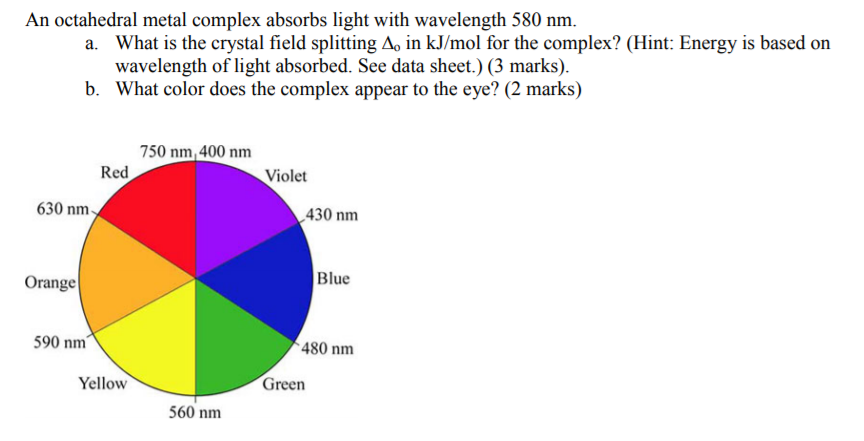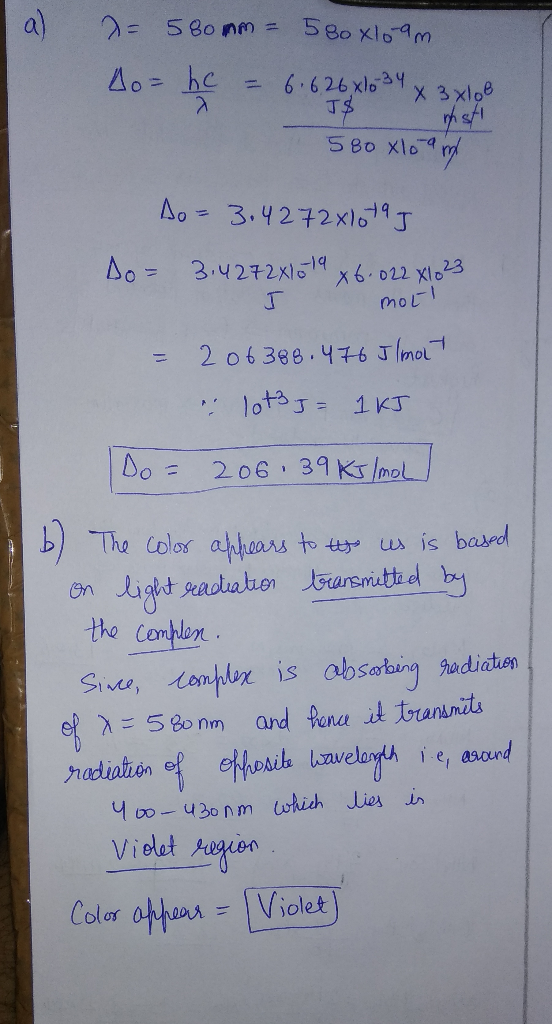#### Earn Coins

Coins can be redeemed for fabulous gifts.

Similar Homework Help Questions
• ### 620 nm Estimate the wavelength of maximum absorption for the octahedral ion trioxalatochromate(III) from the fact...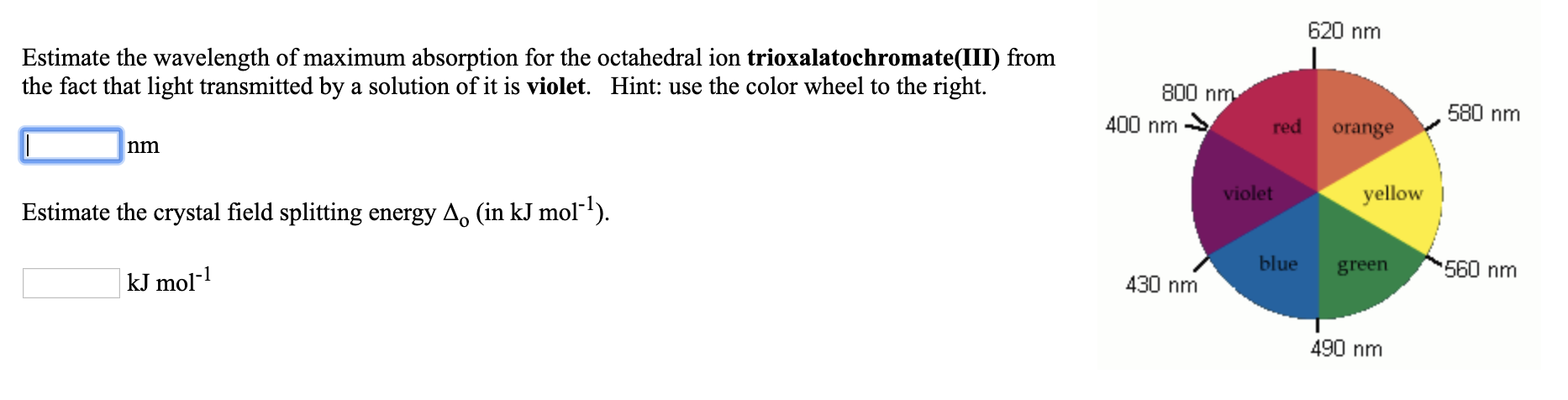620 nm Estimate the wavelength of maximum absorption for the octahedral ion trioxalatochromate(III) from the fact that light transmitted by a solution of it is violet. Hint: use the color wheel to the right. 800 nm 400 nm → red orange 580 nm violet yellow Estimate the crystal field splitting energy A. (in kJ mol-?). blue green kJ mol-1 560 nm 430 nm 490 nm

• ### 13. An octahedral transition metal complex with a d' electron configuration absorbs light of wavelength equal to 650 nm. a. what is the magnitude of the octahedral crystal field splitting par...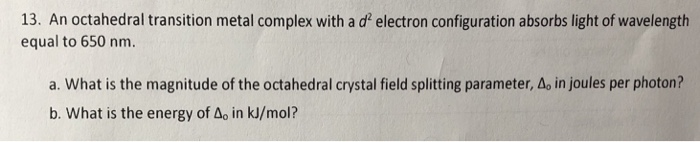13. An octahedral transition metal complex with a d' electron configuration absorbs light of wavelength equal to 650 nm. a. what is the magnitude of the octahedral crystal field splitting parameter, Δο in joules per photon? b. What is the energy of Ao in kJ/mol? 13. An octahedral transition metal complex with a d' electron configuration absorbs light of wavelength equal to 650 nm. a. what is the magnitude of the octahedral crystal field splitting parameter, Δο in joules per...

• ### If [Cr(H2O).]3+ is violet and [Cr(NH3)6]3+ is yellow, which one of these statements is false ?...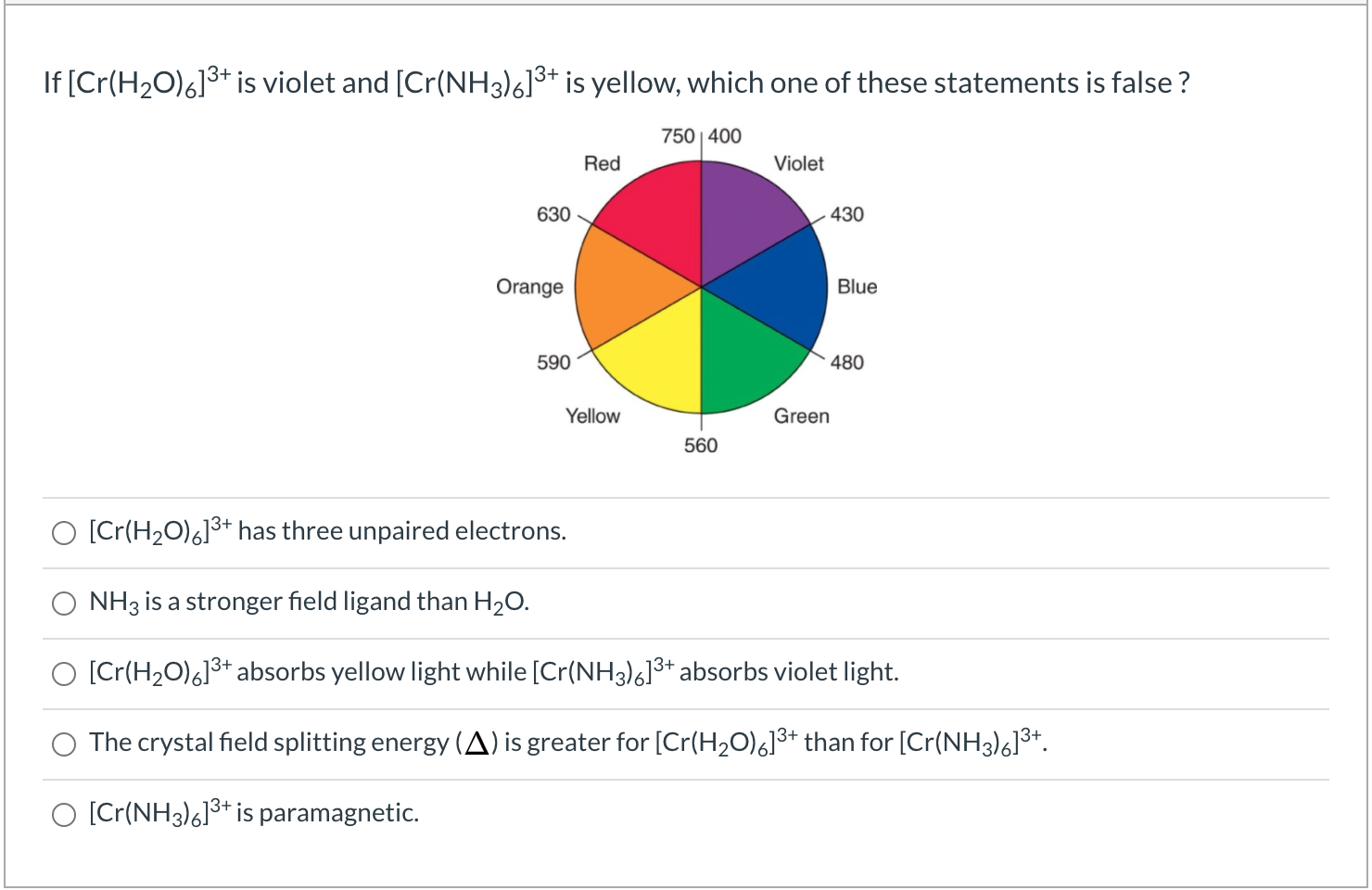If [Cr(H2O).]3+ is violet and [Cr(NH3)6]3+ is yellow, which one of these statements is false ? 750 400 Red Violet 630 430 Orange Blue 590 480 Yellow Green 560 O [Cr(H20)6]3+ has three unpaired electrons. O NH3 is a stronger field ligand than H20. O [Cr(H20)6]3+ absorbs yellow light while [Cr(NH3)6]3+ absorbs violet light. The crystal field splitting energy (A) is greater for [Cr(H20).]3+ than for [Cr(NH3)613+. O [Cr(NH3)613+ is paramagnetic.

• ### References Use the References to access important values if needed for this question. 620 nm Estimate...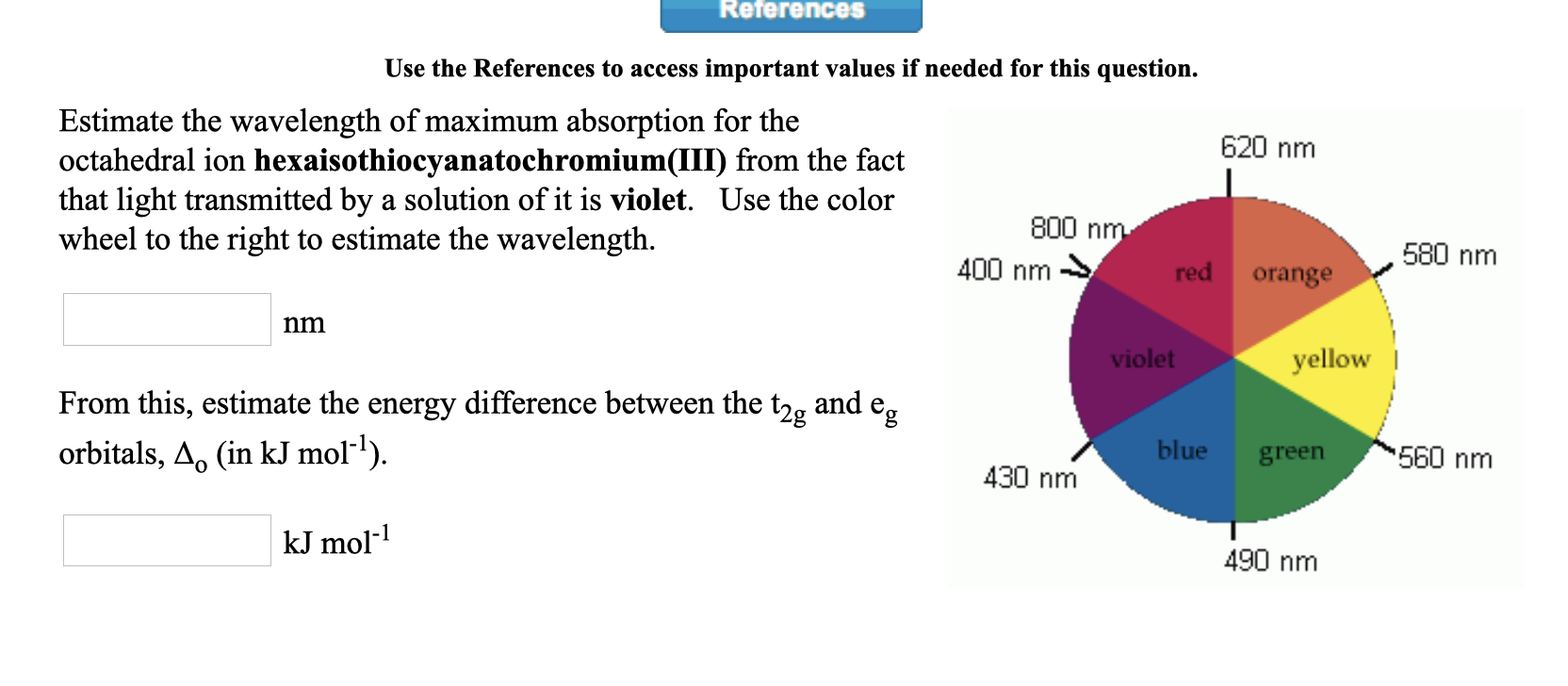References Use the References to access important values if needed for this question. 620 nm Estimate the wavelength of maximum absorption for the octahedral ion hexaisothiocyanatochromium(III) from the fact that light transmitted by a solution of it is violet. Use the color wheel to the right to estimate the wavelength. 800 nm 400 nm red orange 580 nm nm violet yellow From this, estimate the energy difference between the t2, and e, orbitals, A. (in kJ mol-?). blue green 560...

• ### Use the References to access important values if needed for this question. Estimate the wavelength of...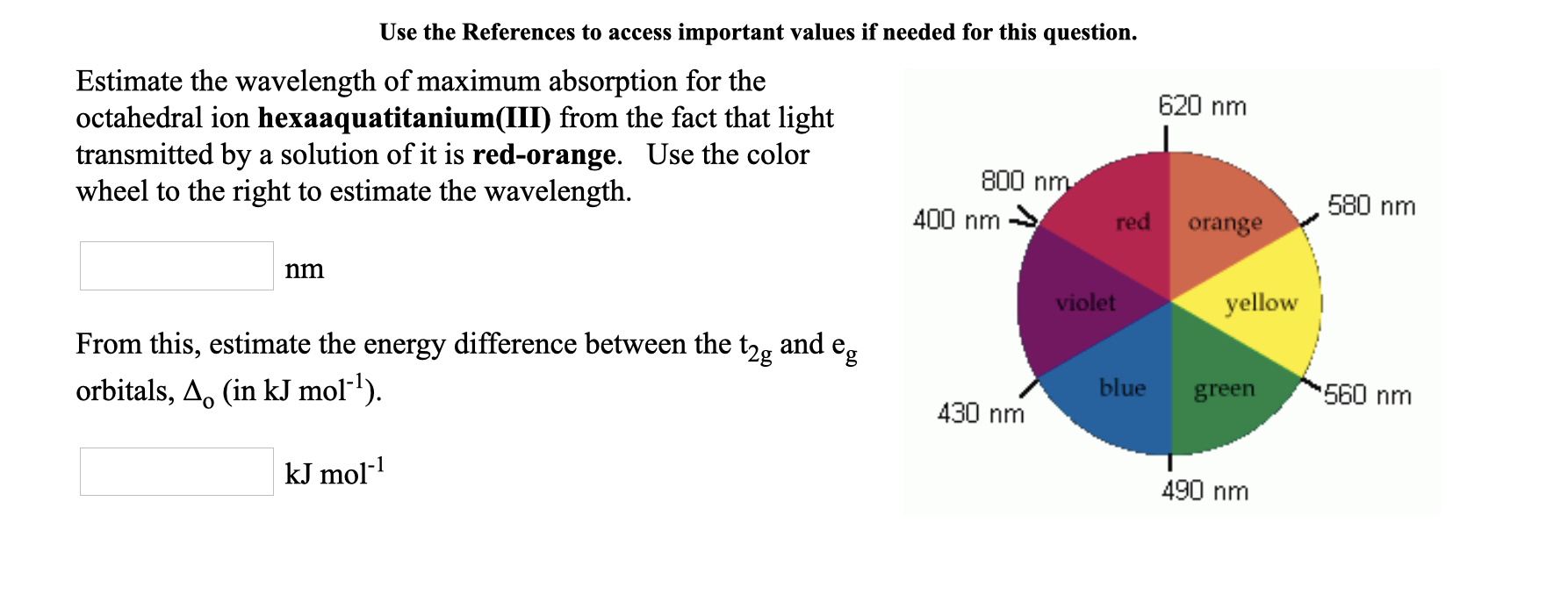Use the References to access important values if needed for this question. Estimate the wavelength of maximum absorption for the 620 nm octahedral ion hexaaquatitanium(III) from the fact that light transmitted by a solution of it is red-orange. Use the color 800 nm wheel to the right to estimate the wavelength. 400 nm → red orange ,580 nm nm violet yellow From this, estimate the energy difference between the t2g and en orbitals, A. (in kJ mol-?). blue green 560...

• ### Please explain any background knowledge and approach to this problem! Very confused about this, my answers are all inco...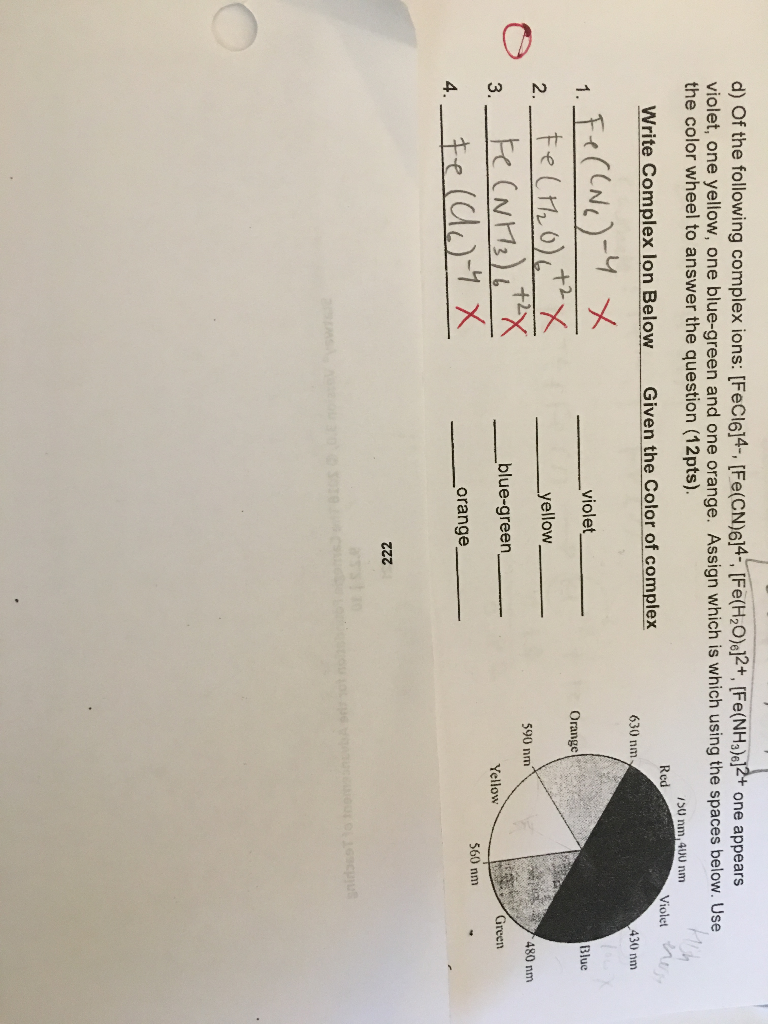Please explain any background knowledge and approach to this problem! Very confused about this, my answers are all incorrect and I do not have a key! d) Of the following complex ions: [FeCl614-, [Fe(CN)6]4-, [Fe(H2O)e12+, [Fe(NH3)e]2+ one appears violet, one yellow, one blue-green and one orange. Assign which is which using the spaces below. Use the color wheel to answer the question (12pts). 1S0 nm,400 nm Red Violet Write Complex lon Below Given the Color of complex 630 nm 430...

• ### Estimate the wavelength of maximum absorption for the octahedral ion hexaamminecobalt(lII) from right the fact that...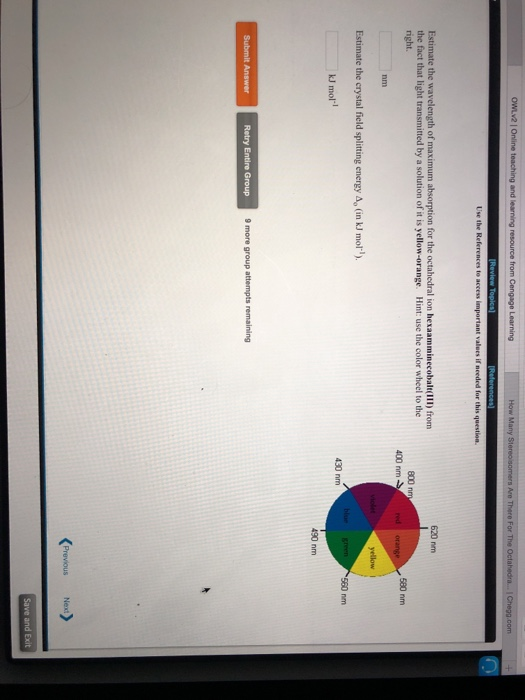Estimate the wavelength of maximum absorption for the octahedral ion hexaamminecobalt(lII) from right the fact that light transmitted by a solution of it is yellow-orange. Hint: use the color wheel to the 620 nm 800 nm 400 nm 580 nm nm Estimate the crystal field splitting energy Ap (in kJ mol) kJ mol-l 430 nm 490 nm Retry Entire Group 9 more group attempts remaining

• ### Question 10 12 pts A) What is the name of the compound having the formula Naz[Fe(NH3)2Br2CICN)...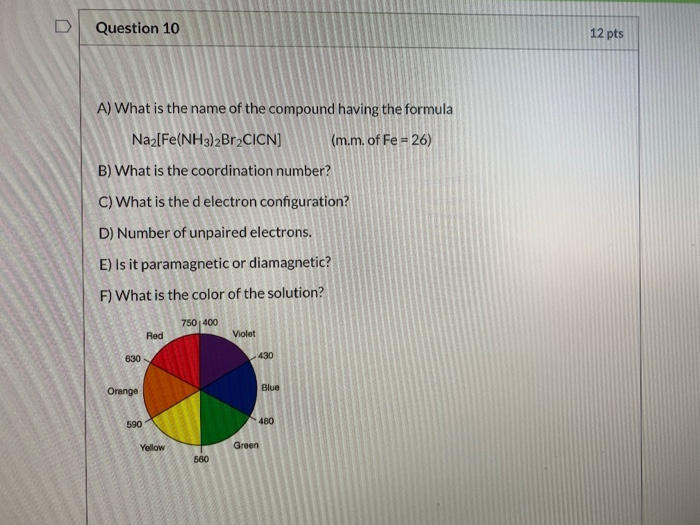Question 10 12 pts A) What is the name of the compound having the formula Naz[Fe(NH3)2Br2CICN) (m.m. of Fe = 26) B) What is the coordination number? C) What is the d electron configuration? D) Number of unpaired electrons. E) Is it paramagnetic or diamagnetic? F) What is the color of the solution? 750 400 Red Violet 630 430 Blue Orange 590 480 Yellow Green 560

• ### 1.. As the wavelength of light increases, the frequency of light Group of answer choices a....

1.. As the wavelength of light increases, the frequency of light Group of answer choices a. Stays the same b. increases c. decreases 2.. A typical AM radio station broadcasts at a frequency of 1070. MHz. What is the wavelength of the electromagnetic radiation generated by this radio station? 1 Hz = 1 s-1 1 MHz = 106 Hz Group of answer choices a. 3.21 x 10^11 m b. 2.804 X 10 ^5 m c. 0.2804 m 3.. Use this...

• ### CE IZOLIUM The (RhC16]has its maximum absorbance at 495 nm. 1. Calculate the crystal field splitting...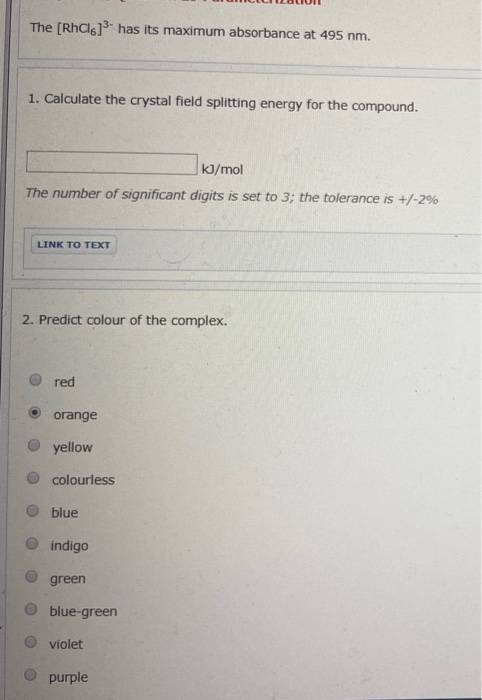CE IZOLIUM The (RhC16]has its maximum absorbance at 495 nm. 1. Calculate the crystal field splitting energy for the compound. kJ/mol The number of significant digits is set to 3; the tolerance is +/-2% LINK TO TEXT 2. Predict colour of the complex. red orange yellow O colourless O blue indigo O green blue-green violet O purple